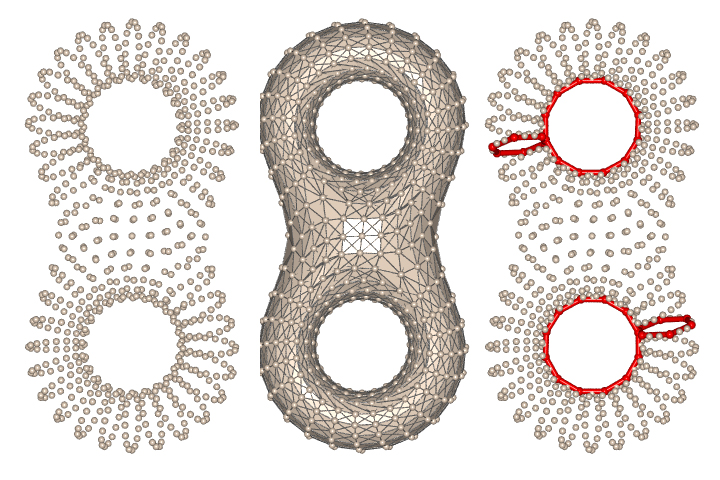CS 59300-TDA: Computational Topology for Data Analysis

Tamal K Dey

Home
Research pages(TBA)
List of Papers (partially constructed)
Class Schedule

Time: SP 2023, TTh 1:30--2:45pm

Computational topology has played a synergistic role in bringing together research work from computational geometry, algebraic topology, data analysis, and many  other related scientific areas. In recent years, the field has undergone particular growth in the area of data analysis. The application of topological techniques to traditional data analysis, which before has mostly developed on a statistical setting, has opened up new opportunities. This course is intended to cover this aspect of computational topology along with the developments of generic techniques for various topology-centered problems.

• Objectives
• Be familiar with basics in topology that are useful for computing with data
• Master a subset of algorithms for computing: Betti number, topological persistence, homology cycles, Reeb graphs, discrete Morse structures, multiparameter persistence
• Be familiar with how to design algorithms for problems in applications dealing with data
• Be familiar with how to research the background of a topic in topological data analysis, machine learning
• Prerequisites: Grad standing and permission of instructor
• Materials
•  Text and class material 1. Computational topology, Herbert Edelsbrunner and John L. Harer, AMS 2. Curve and surface reconstruction: Algorithms with mathematical analysis, Tamal K. Dey, Cambridge U. Press 3. Elements of Algebraic Topology, James R. Munkres, Addison-Wesley 4. Algebraic Topology, Allen Hatcher, Cambridge U. Press 5. Class materials and notes posted on this web-site Topics 1. Basics Topology These notes are much shortened versions of some chapters from an upcoming book ``Computational Topology for Data Analysis" by myself and Y. Wang (UC SanDiego) to be published by Cambridge U. Press Go here for the FRREE ELECTRONIC copy of the entire book. a. Topological spaces, metric space topology [Notes] b. Maps: homeomorphisms, homotopy equivalence, isotopy [Notes] c. Manifolds [Notes] [ClassScribble1] [ClassScribble2] [ClassScribble3] 2. Complexes on data a. Simplicial complexes [Munkres][Notes] b. Chech complexes, Vietoris-Rips complexes [Notes] c. Witness complexes [deSilva-Carlsson04 paper][Notes] d. Graph induced complexes  [DeyFanWang13 paper][Notes] [ClassScribble] 3. Homology a. Chains, boundaries, homology groups, betti numbers [Notes, Munkres book] c. Induced maps among homology groups [Notes, Munkres book] d. Singular homology groups [Notes, Munkres book] f. Cohomology groups [Notes, Hatcher book] [ClassScribble] 4. Topological persistence a. Filtrations, Persistent homology [Notes] b. Persistence diagram [Notes]  Cohen-SteinerEdelsbrunnerHarer07 paper proves the stabilty of persistence c. Persistence algorithm [Notes] [C-VII Edelsbrunner-Harer book, EdelsbrunneLetscherZomorodian02 paper introduced topological persistence, ZomorodianCarlsson04 paper brings algebra into persistence] [ClassScribble1] [ClassScribble2] 5. General Persistence (Zigzag) a. Towers, Persistence modules from simplicial maps[Notes] b. Algorithm for towers [Notes] [DeyFanWang13SM paper on Annotations] c. Zigzag persistence and algorithms [Notes] [CarlssonSilvaMorozov09 paper on zigzag persistenc][DeyHou latest algo(2022)] d. Level Set persistence [Notes] e. Extended persistence [Notes, see the book] [ClassScribble1][ClassScribble2] 6. Generators and Otimality a. Computing optimal cycle basis [Notes] [EricksonWhittlesey05 paper on greedy basis construction and b. Presentation slides, DeySunWang09 paper on shortest basis from point data] c. Optimizing within a class [Notes][Presentation slides, DeyHiraniKrishnamoorthy10 paper on LP algorithm for shortest homologous cycle] d. Computing optimal persistent cycles [Notes] [DeyHouMandal 2020 SODA paper] 7. Topology inference from point cloud data a. Computing homology from data [Notes, ChazalOudot08 paper on homology inference, CCGGO09 paper on interleaving of persistence modules] b. Sparsification to handle big data [Notes] [,  [Sheehy12 paper on sparsified Rips complex, DeyFanWang13 paper on subsampling] c. Homology inference [Notes] d. Persistence diagram approximation from scalar data[Notes] 8. Persistence on graphs and Reeb graphs a. Reeb graphs [Notes] b. Interleaving distance [Notes] c. Comparing graphs with persistence summary [Notes] 9. Discrete Morse Theory and Persistence a. Discrete Morse [Notes] b. Discrete Morse Vector Field (DMVF) [Notes] c. Persistence Based DMVF [Notes] d. Application to graph reconstruction [Notes] 10. Nerves, Mapper, Multiscale Mapper a. Nerves [Notes] b. Mapper [Notes] c. Multiscale mapper [Notes] 11. Multiparameter persistence module decomposition a. Multiparameter persistence [Notes] b. Computing indecomposables [Notes] c. Invariants [Notes] 12. Multiparameter persistence and distances a. Multiparameter persistence module from categorial viewpoint [Notes] b. Computing matching distance [Notes] c. Computing interleaving distance [Notes]